# Differentiating Inverse Functions

## Inverse Function Review

One application of the chain rule is to compute the derivative of an inverse function. First, let's review the definition of an inverse function:

We say that the function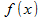is invertible on an interval [a, b] if there are no pairs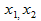in the interval such that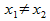and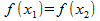. That means there are no two x-values that have the same y-value. That's important, because if two x-coordinates map to the same y-coordinate, the inverse function (working in reverse) would map a single x-coordinate to multiple y-coordinates. That doesn't make sense, because f(x) could have more than one resulting value!

We say that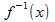is the inverse of an invertible functionon [a, b] if: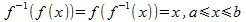For example, the functions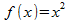and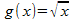are inverses on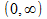since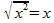on that interval. Note that it works both ways -- the inverse function of the original function returns x, and the original function performed on the inverse ALSO returns x.

### Taking The Derivative

So, how do we differentiate an inverse function? Recall the chain rule: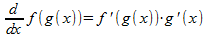Applying this to the definition of an inverse function, we have: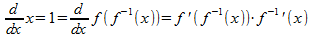So: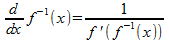Let's see how to apply this to real examples.

#### Example 1

Letso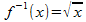as above. Then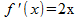, and applying the formula we have: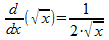This agrees with the answer we would get from viewing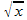as the polynomial function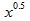.

#### Example 2

The function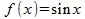is invertible on the interval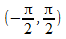, with inverse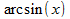. We know that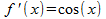, so applying our formula we see that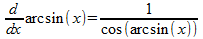We can check that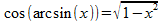, which means that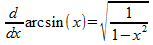.